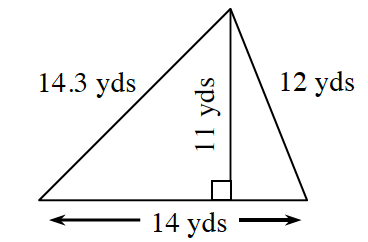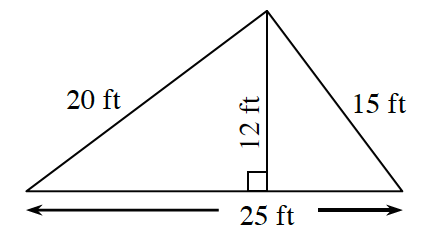### Home > CC2MN > Chapter 7 > Lesson 7.2.2 > Problem7-69

7-69.

Find the perimeter and area of each triangle below.

1.The perimeter is the sum of all the sides of the shape.

$14.3+12+14=\text{perimeter}$

Remember to include units.

The area of a triangle can be found using the formula:

$\text{Area} =\frac{1}{2}\text{(base)(height)}$

Remember to include units.

$\text{Area}=\frac{1}{2}(14)(11)$

1.See part (a).